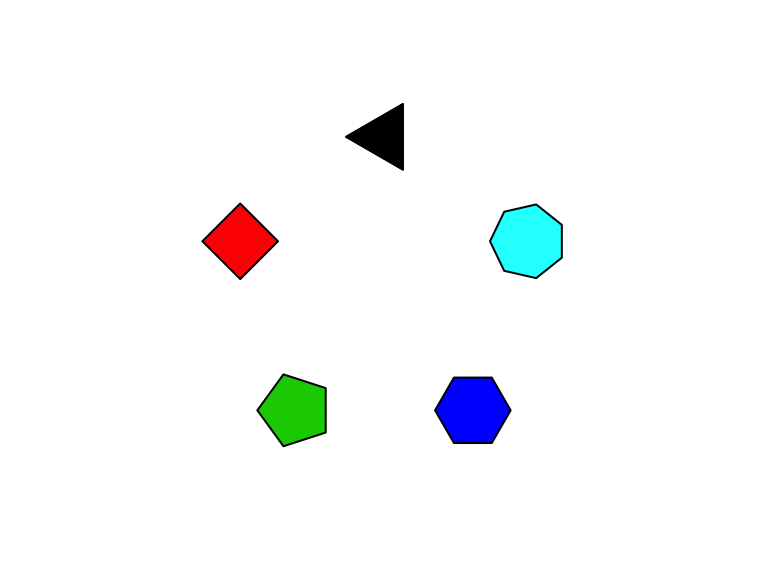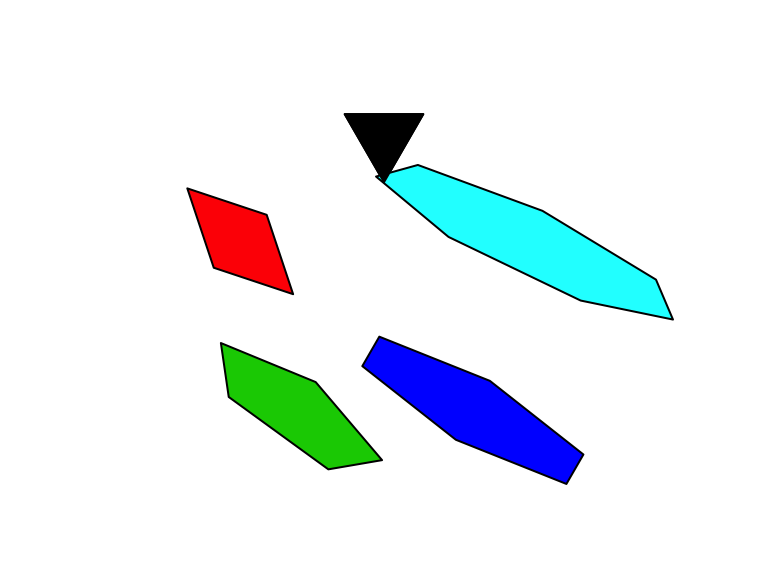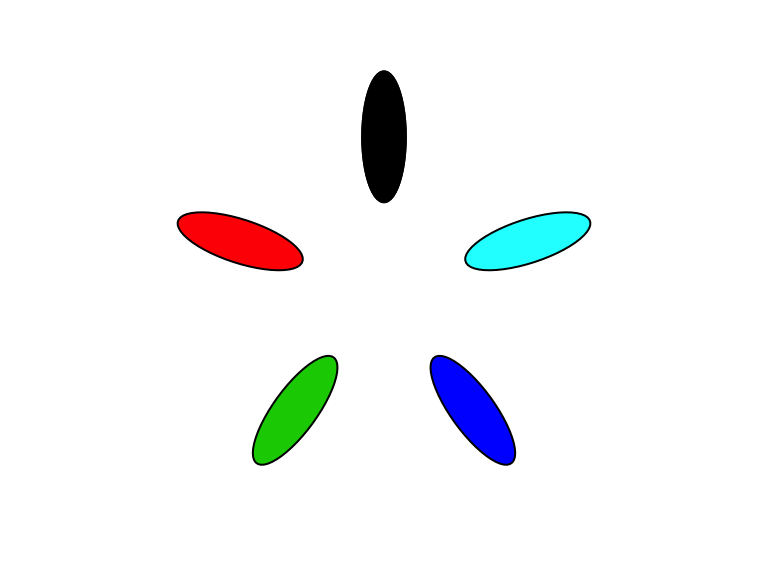# Regular polygons and ellipses in grid graphics

#### 2017-09-09

The gridExtra package provides a basic implementation of regular polygons, ngonGrob()/grid.ngon, and a convenience function to draw ellipses, ellipseGrob()/grid.ellipse(). We illustrate below the basic usage of these vectorised functions.

## Basic usage

library(gridExtra)
library(grid)
library(grid)
N <- 5
xy <- polygon_regular(N)*2

# draw multiple polygons
g <- ngonGrob(unit(xy[,1],"cm") + unit(0.5,"npc"),
unit(xy[,2],"cm") + unit(0.5,"npc"),
n=seq_len(N)+2, gp=gpar(fill=1:N))

grid.newpage()
grid.draw(g)## Rotated and stretched polygons

g2 <- ngonGrob(unit(xy[,1],"cm") + unit(0.5,"npc"),
unit(xy[,2],"cm") + unit(0.5,"npc"),
n=seq_len(N)+2, ar=seq_len(N),
phase=0, angle=pi/(seq_len(N)+2),
size=1:N+5, gp=gpar(fill=1:N))

grid.newpage()
grid.draw(g2)## Ellipses

g3 <- ellipseGrob(unit(xy[,1],"cm") + unit(0.5,"npc"),
unit(xy[,2],"cm") + unit(0.5,"npc"),
angle=-2*seq(0,N-1)*pi/N+pi/2,
size=5, ar=3, gp=gpar(fill=1:N))

grid.newpage()
grid.draw(g3)